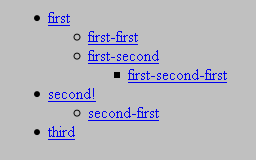# Copy & Replace¶

These node operations are less frequently used than all the others, but nevertheless useful. Common use cases are content picking or building trees.

`→ node.copy()` deep-copies the node in its current state for later re-usage. `→ node.replace()` replaces a node with a copy of another node.

## Content Picking With `node.replace()`¶

The following may look strange, but it’s a very elegant method to put content of similar frames into one template and pick the one you want to display now.

```from tdi import NodeNotFoundError
from tdi import html
template = html.from_string("""
<html>
<body>
<div tdi="content">
<div tdi="page_1">
This is page 1
</div>
<div tdi="page_2">
This is page 2
</div>
</div>
</body>
</html>
""")

class Model(object):
def __init__(self, page):
self._page = page

def render_content(self, node):
try:
page, number = node("page_%s" % self._page), self._page
except NodeNotFoundError:
page, number = node.page_1, 1
node.replace(self.render_page, page, number)

def render_page(self, node, page_no):
node['title'] = u"Page %s" % page_no

model = Model(page=2)
template.render(model)
model = Model(page=10)
template.render(model)
```

The principle is simple. A parent node is replaced by one of its child nodes. That way, all the other child nodes simply vanish. This is possible, because `→ node.replace()` first copies the replacing node.

```<html>
<body>
<div title="Page 2">
This is page 2
</div>
</body>
</html>

<html>
<body>
<div title="Page 1">
This is page 1
</div>
</body>
</html>
```

This is just one use case for replacing nodes. There are many others. For example, you can replace a node by itself and just place a callback function. This is useful for example, if you want to split the logic for some reason. Also, these callbacks are executed, even if the node is contained in a subtree marked as “done”.

Note that you don’t need to pass a render callback at all (pass `None` then).

`→ node.replace()` both modifies the node in-place and also conveniently returns the node again.

## Building Trees With `node.copy()` And `node.replace()`¶

The `→ copy` and `→ replace` methods are the basic building blocks for unlimited content nesting, like building trees. Here’s a simplified example:

```from tdi import html
template = html.from_string("""
<html>
<body>
<ul tdi="tree">
<tdi tdi="*-next" />
</li>
</ul>
</body>
</html>
""")

class Model(object):
def __init__(self, tree):
self._tree = tree

def render_tree(self, node):
tree_node = node.copy()
def level(node, (title, tree)):
if tree:
node.next.replace(None, tree_node).item.repeat(level, tree)
node.item.repeat(level, self._tree)

tree = (
(u'first', (
(u'first-first', ()),
(u'first-second', (
(u'first-second-first', ()),
)),
)),
(u'second!', (
(u'second-first', ()),
)),
(u'third', ()),
)
model = Model(tree=tree)
template.render(model)
```

The tree is defined in this example as nested tuples. Every level is rendered into an unordered list (`<ul>`). The prototype of such a list (the `<ul>` node named `tree`) is initially copied and stored. The children of each current level are passed as iterable to the `→ repeat` method along with the `level` callback which does the actual work until no more children are available. This work consists of filling the currently rendered item and placing the prototype at the place of the `next` node, along with a new `repeat()` method call. The whole chain is bootstrapped by the `node.item.repeat()` call at the end of the `render_tree()` method.

Note that while the definition looks recursive, the execution is not recursive, because the `repeat()` method just marks the node for repetition. TDI picks it up at the time the node is actually rendered. Every single repeat operation is executed lazily on demand, so deep nesting levels don’t hurt in any way.

Finally, here’s the result emitted by the script:

```<html>
<body>
<ul>
<li><a href="/first/">first</a>
<ul>
<li><a href="/first-first/">first-first</a>

</li><li><a href="/first-second/">first-second</a>
<ul>
<li><a href="/first-second-first/">first-second-first</a>

</li>
</ul>
</li>
</ul>
</li><li><a href="/second!/">second!</a>
<ul>
<li><a href="/second-first/">second-first</a>

</li>
</ul>
</li><li><a href="/third/">third</a>

</li>
</ul>
</body>
</html>
```

Since the resulting HTML is not very pretty, here’s the result as shown by a browser:The tree rendered by a browser